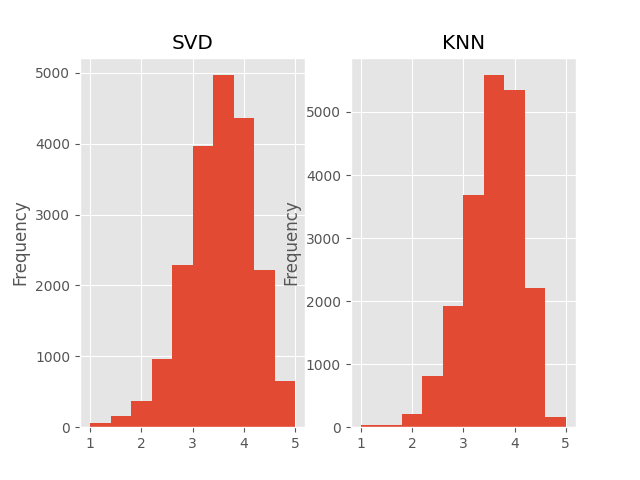# Comparison of two algorithms¶

We will see in this notebook how we can compare the prediction accuracy of two algorithms.

In :
from __future__ import (absolute_import, division, print_function,
unicode_literals)
import pickle
import os

import pandas as pd

from surprise import SVD
from surprise import KNNBasic
from surprise import Dataset
from surprise.model_selection import PredefinedKFold
from surprise import dump
from surprise.accuracy import rmse

In [ ]:
# We will train and test on the u1.base and u1.test files of the movielens-100k dataset.
# You can do it manually, or by running:

# Now, let's load the dataset
train_file = os.path.expanduser('~') + '/.surprise_data/ml-100k/ml-100k/u1.base'
test_file = os.path.expanduser('~') + '/.surprise_data/ml-100k/ml-100k/u1.test'

pkf = PredefinedKFold()

# We'll use the well-known SVD algorithm and a basic nearest neighbors approach.
algo_svd = SVD()
algo_knn = KNNBasic()

for trainset, testset in pkf.split(data):
algo_svd.fit(trainset)
predictions_svd = algo_svd.test(testset)

algo_knn.fit(trainset)
predictions_knn = algo_knn.test(testset)

rmse(predictions_svd)
rmse(predictions_knn)

dump.dump('./dump_SVD', predictions_svd, algo_svd)
dump.dump('./dump_KNN', predictions_knn, algo_knn)

Computing the msd similarity matrix...
Done computing similarity matrix.

In :
# The dumps have been saved and we can now use them whenever we want.

df_svd = pd.DataFrame(predictions_svd, columns=['uid', 'iid', 'rui', 'est', 'details'])
df_knn = pd.DataFrame(predictions_knn, columns=['uid', 'iid', 'rui', 'est', 'details'])

df_svd['err'] = abs(df_svd.est - df_svd.rui)
df_knn['err'] = abs(df_knn.est - df_knn.rui)


We now have two dataframes with the all the predictions for each algorithm. The cool thing is that, as both algorithm have been tested on the same testset, the indexes of the two dataframes are the same!

In :
df_svd.head()

Out:
uid iid rui est details err
0 184 67 3.0 3.070263 {'was_impossible': False} 0.070263
1 766 487 3.0 3.797903 {'was_impossible': False} 0.797903
2 263 117 3.0 3.594508 {'was_impossible': False} 0.594508
3 545 168 4.0 3.961151 {'was_impossible': False} 0.038849
4 525 255 1.0 3.306502 {'was_impossible': False} 2.306502
In :
df_knn.head()

Out:
uid iid rui est details err
0 184 67 3.0 3.043189 {'actual_k': 40, 'was_impossible': False} 0.043189
1 766 487 3.0 4.139804 {'actual_k': 40, 'was_impossible': False} 1.139804
2 263 117 3.0 3.525691 {'actual_k': 40, 'was_impossible': False} 0.525691
3 545 168 4.0 4.393259 {'actual_k': 40, 'was_impossible': False} 0.393259
4 525 255 1.0 3.638801 {'actual_k': 40, 'was_impossible': False} 2.638801
In :
# Let's check how good are the KNN predictions when the SVD has a huge error:
df_knn[df_svd.err >= 3.5]

Out:
uid iid rui est details err
533 405 452 5.0 2.370203 {'actual_k': 40, 'was_impossible': False} 2.629797
1557 295 183 1.0 4.275709 {'actual_k': 40, 'was_impossible': False} 3.275709
4431 481 318 1.0 4.855612 {'actual_k': 40, 'was_impossible': False} 3.855612
6579 405 1218 5.0 3.329299 {'actual_k': 21, 'was_impossible': False} 1.670701
10032 239 514 1.0 4.250013 {'actual_k': 40, 'was_impossible': False} 3.250013
14311 425 313 1.0 4.093898 {'actual_k': 40, 'was_impossible': False} 3.093898
15979 405 1053 5.0 3.497124 {'actual_k': 17, 'was_impossible': False} 1.502876
19292 1 131 1.0 3.779858 {'actual_k': 40, 'was_impossible': False} 2.779858
In :
# Well... Not much better.
# Now, let's look at the predictions of SVD on the 10 worst predictions for KNN
df_svd.iloc[df_knn.sort_values(by='err')[-10:].index]

Out:
uid iid rui est details err
14619 771 98 1.0 4.153106 {'was_impossible': False} 3.153106
1557 295 183 1.0 4.632378 {'was_impossible': False} 3.632378
11759 405 1405 1.0 1.915805 {'was_impossible': False} 0.915805
4493 181 1242 1.0 2.277950 {'was_impossible': False} 1.277950
10970 279 1242 1.0 3.515677 {'was_impossible': False} 2.515677
2657 239 318 1.0 4.144616 {'was_impossible': False} 3.144616
4431 481 318 1.0 4.580412 {'was_impossible': False} 3.580412
12838 167 1306 5.0 3.136852 {'was_impossible': False} 1.863148
16681 288 1358 5.0 3.253280 {'was_impossible': False} 1.746720
12869 363 1512 1.0 3.425335 {'was_impossible': False} 2.425335
In :
# How different are the predictions from both algorithms ?
# Let's count the number of predictions for each rating value

import matplotlib.pyplot as plt
import matplotlib
%matplotlib notebook
matplotlib.style.use('ggplot')

figure, (ax1, ax2) = plt.subplots(1, 2)

df_svd.est.plot(kind='hist', title='SVD', ax=ax1)
df_knn.est.plot(kind='hist', title='KNN', ax=ax2)

# As expected, one of the drawbacks of the NN algorithms is that their predictions are often
# quite concentrated around the mean. The SVD algorithm seems more confortable predicting extreme rating values.Out:
<matplotlib.axes._subplots.AxesSubplot at 0x7f073c40a760>
In :
# Question: when a user has rated only a small number of items (less than 10), which algorithm
# gives the best predictions on average?

def get_Iu(uid):
"""Return the number of items rated by given user

Args:
uid: The raw id of the user.
Returns:
The number of items rated by the user.
"""

try:
return len(trainset.ur[trainset.to_inner_uid(uid)])
except ValueError:  # user was not part of the trainset
return 0

df_knn['Iu'] = df_knn.uid.apply(get_Iu)
df_svd['Iu'] = df_svd.uid.apply(get_Iu)

df_knn[df_knn.Iu < 10].err.mean(), df_svd[df_svd.Iu < 10].err.mean()

Out:
(nan, nan)
In [ ]: